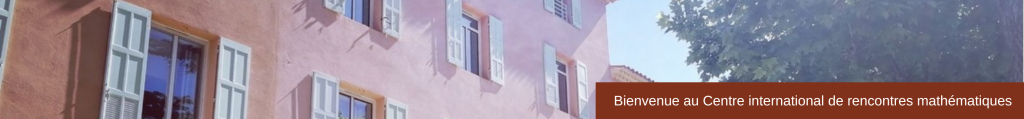RESEARCH IN PAIRS
 The Multiplicative Ergodic Theorem for Stochastic Differential Equations with Fractional NoiseThéorème ergodique multiplicatif pour les équations différentielles stochastiques avec bruit fractionnel​15 – 26 March 2021
Description
The Multiplicative Ergodic Theorem (MET), established originally by Valery Oseledets in 1968, describes the dynamics of linear Random Dynamical Systems (RDS). This theorem allows to split the phase space into invariant random subspaces, along which the system has a particular asymptotical exponential growth rate, in the sense that all elements (except the origin) in each subspace generate the same Lyapunov exponent. The goal of this project is to apply the MET to differential equations that are driven by fractional Brownian motions (fBm) B^H with Hurst parameter H in (0,1). It is well-known that, despite the fact that the behavior of such noises differ so much from the standard Brownian motion, the fBm generates an ergodic metric dynamical system. For H>1/2 the stochastic integral appearing in the SDE is either presented by the Young integral or by fractional derivatives. In the case H in (1/3,1/2], the stochastic integral can be interpreted in the sense of rough paths or in the sense of compensated fractional derivatives. No matter the range of the Hurst parameter, a qualitative difference to the classical SDE is that the stochastic integrals appearing in these equations are pathwise defined, which in particular exclude possible troubles regarding the anticipativity of initial conditions in the classical stochastic integration. Assuming that the system provides the linear stability structure of the MET, we can then deal with several applications: (locally) stable, unstable and centered random manifolds of nonlinear equations with domain given by the Oseledets spaces. Another interesting and challenging objective is to investigate the application of the MET to linear stochastic evolution equations in Hilbert spaces.
Participants

María J. Garrido-Atienza (University of Seville)
Björn Schmalfuß (Friedrich Schiller University of Jena)